Note: The other languages of the website are Google-translated. Back to English

## 如何在Excel中將數字轉換為印度盧比的單詞？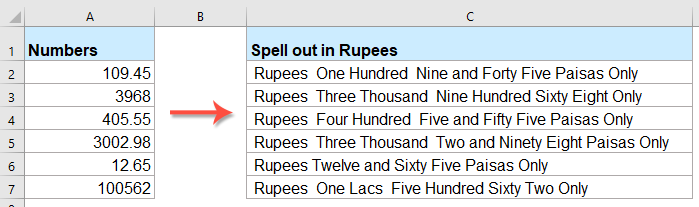#### 使用VBA代碼將數字轉換為印度盧比的單詞

1。 按住 ALT + F11 鍵打開 Microsoft Visual Basic for Applications 窗口。

2。 點擊 插入 > 模塊，然後將以下代碼粘貼到“模塊窗口”中。

VBA代碼：將數字轉換為盧比的單詞

``````Public Function RupeeFormat(SNum As String)
'Updateby Extendoffice
Dim xDPInt As Integer
Dim xArrPlace As Variant
Dim xRStr_Paisas As String
Dim xNumStr As String
Dim xF As Integer
Dim xTemp As String
Dim xStrTemp As String
Dim xRStr As String
Dim xLp As Integer
xArrPlace = Array("", "", " Thousand ", " Lacs ", " Crores ", " Trillion ", "", "", "", "")
On Error Resume Next
If SNum = "" Then
RupeeFormat = ""
Exit Function
End If
xNumStr = Trim(str(SNum))
If xNumStr = "" Then
RupeeFormat = ""
Exit Function
End If

xRStr = ""
xLp = 0
If (xNumStr > 999999999.99) Then
RupeeFormat = "Digit excced Maximum limit"
Exit Function
End If
xDPInt = InStr(xNumStr, ".")
If xDPInt > 0 Then
If (Len(xNumStr) - xDPInt) = 1 Then
xRStr_Paisas = RupeeFormat_GetT(Left(Mid(xNumStr, xDPInt + 1) & "0", 2))
ElseIf (Len(xNumStr) - xDPInt) > 1 Then
xRStr_Paisas = RupeeFormat_GetT(Left(Mid(xNumStr, xDPInt + 1), 2))
End If
xNumStr = Trim(Left(xNumStr, xDPInt - 1))
End If
xF = 1
Do While xNumStr <> ""
If (xF >= 2) Then
xTemp = Right(xNumStr, 2)
Else
If (Len(xNumStr) = 2) Then
xTemp = Right(xNumStr, 2)
ElseIf (Len(xNumStr) = 1) Then
xTemp = Right(xNumStr, 1)
Else
xTemp = Right(xNumStr, 3)
End If
End If
xStrTemp = ""
If Val(xTemp) > 99 Then
xStrTemp = RupeeFormat_GetH(Right(xTemp, 3), xLp)
If Right(Trim(xStrTemp), 3) <> "Lac" Then
xLp = xLp + 1
End If
ElseIf Val(xTemp) <= 99 And Val(xTemp) > 9 Then
xStrTemp = RupeeFormat_GetT(Right(xTemp, 2))
ElseIf Val(xTemp) < 10 Then
xStrTemp = RupeeFormat_GetD(Right(xTemp, 2))
End If
If xStrTemp <> "" Then
xRStr = xStrTemp & xArrPlace(xF) & xRStr
End If
If xF = 2 Then
If Len(xNumStr) = 1 Then
xNumStr = ""
Else
xNumStr = Left(xNumStr, Len(xNumStr) - 2)
End If
ElseIf xF = 3 Then
If Len(xNumStr) >= 3 Then
xNumStr = Left(xNumStr, Len(xNumStr) - 2)
Else
xNumStr = ""
End If
ElseIf xF = 4 Then
xNumStr = ""
Else
If Len(xNumStr) <= 2 Then
xNumStr = ""
Else
xNumStr = Left(xNumStr, Len(xNumStr) - 3)
End If
End If
xF = xF + 1
Loop
If xRStr = "" Then
xRStr = "No Rupees"
Else
xRStr = " Rupees " & xRStr
End If
If xRStr_Paisas <> "" Then
xRStr_Paisas = " and " & xRStr_Paisas & " Paisas"
End If
RupeeFormat = xRStr & xRStr_Paisas & " Only"
End Function
Function RupeeFormat_GetH(xStrH As String, xLp As Integer)
Dim xRStr As String
If Val(xStrH) < 1 Then
RupeeFormat_GetH = ""
Exit Function
Else
xStrH = Right("000" & xStrH, 3)
If Mid(xStrH, 1, 1) <> "0" Then
If (xLp > 0) Then
xRStr = RupeeFormat_GetD(Mid(xStrH, 1, 1)) & " Lac "
Else
xRStr = RupeeFormat_GetD(Mid(xStrH, 1, 1)) & " Hundred "
End If
End If
If Mid(xStrH, 2, 1) <> "0" Then
xRStr = xRStr & RupeeFormat_GetT(Mid(xStrH, 2))
Else
xRStr = xRStr & RupeeFormat_GetD(Mid(xStrH, 3))
End If
End If
RupeeFormat_GetH = xRStr
End Function
Function RupeeFormat_GetT(xTStr As String)
Dim xTArr1 As Variant
Dim xTArr2 As Variant
Dim xRStr As String
xTArr1 = Array("Ten", "Eleven", "Twelve", "Thirteen", "Fourteen", "Fifteen", "Sixteen", "Seventeen", "Eighteen", "Nineteen")
xTArr2 = Array("", "Twenty", "Thirty", "Forty", "Fifty", "Sixty", "Seventy", "Eighty", "Ninety")
Result = ""
If Val(Left(xTStr, 1)) = 1 Then
xRStr = xTArr1(Val(Mid(xTStr, 2, 1)))
Else
If Val(Left(xTStr, 1)) > 0 Then
xRStr = xTArr2(Val(Left(xTStr, 1)) - 1)
End If
xRStr = xRStr & RupeeFormat_GetD(Right(xTStr, 1))
End If
RupeeFormat_GetT = xRStr
End Function
Function RupeeFormat_GetD(xDStr As String)
Dim xArr_1() As Variant
xArr_1 = Array(" One", " Two", " Three", " Four", " Five", " Six", " Seven", " Eight", " Nine", "")
If Val(xDStr) > 0 Then
RupeeFormat_GetD = xArr_1(Val(xDStr) - 1)
Else
RupeeFormat_GetD = ""
End If
End Function
``````

3。 插入代碼後，保存並關閉代碼窗口，返回到工作表，然後輸入以下公式： = RupeeFormat（A2） 到一個空白單元格中，然後向下拖動填充手柄以將此公式應用於其他單元格，所有數字均以盧比表示，請參見屏幕截圖：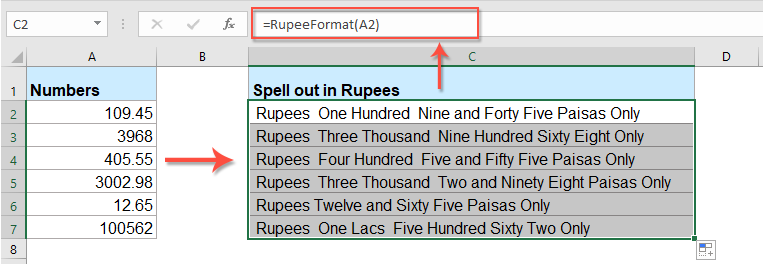#### 具有驚人功能的將數字轉換為英文單詞

1。 選擇要轉換的數字列表，然後單擊 庫工具 > 內容 > 數字到單詞，請參見屏幕截圖：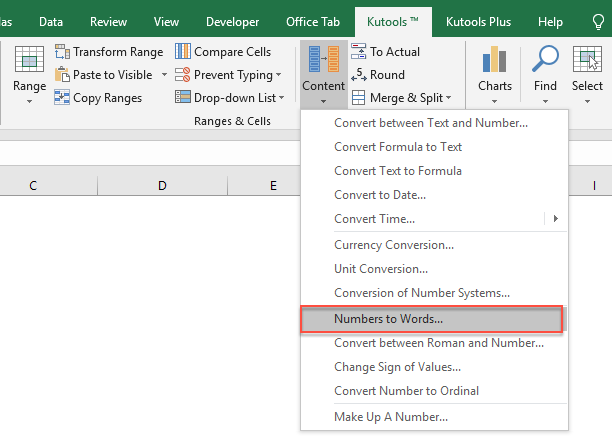2。 在 數字到貨幣單詞 對話框中選擇 English 選項從 語言 部分，然後單擊 Ok 按鈕，所選內容中的數字已轉換為英語貨幣單詞，請參見屏幕截圖：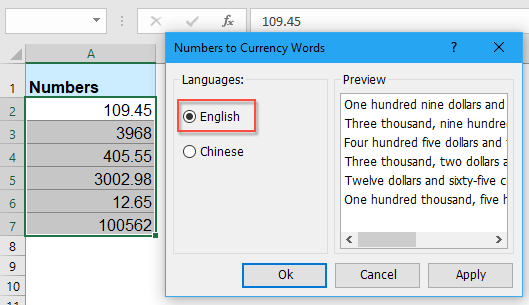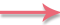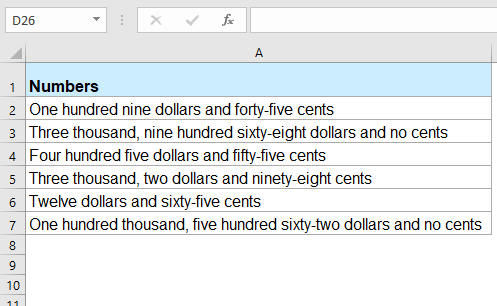## 最佳辦公效率工具

### Kutools for Excel 解決了你的大部分問題，並將你的生產力提高了 80%

• 超級公式欄 （輕鬆編輯多行文本和公式）； 閱讀版式 （輕鬆讀取和編輯大量單元格）； 粘貼到過濾範圍...
• 合併單元格/行/列 和保存數據； 拆分單元格內容； 合併重複的行和總和/平均值...防止細胞重複； 比較範圍...
• 選擇重複或唯一 行; 選擇空白行 （所有單元格都是空的）； 超級查找和模糊查找 在許多工作簿中； 隨機選擇...
• 確切的副本 多個單元格，無需更改公式參考； 自動創建參考 到多張紙； 插入項目符號，複選框等...
• 收藏并快速插入公式，範圍，圖表和圖片； 加密單元 帶密碼 創建郵件列表 並發送電子郵件...
• 提取文字，添加文本，按位置刪除， 刪除空間; 創建和打印分頁小計； 在單元格內容和註釋之間轉換...
• 超級濾鏡 （將過濾方案保存並應用於其他工作表）； 高級排序 按月/週/日，頻率及更多； 特殊過濾器 用粗體，斜體...
• 結合工作簿和工作表; 根據關鍵列合併表； 將數據分割成多個工作表; 批量轉換xls，xlsx和PDF...
• 數據透視表分組依據 週號，週幾等 顯示未鎖定的單元格 用不同的顏色 突出顯示具有公式/名稱的單元格...### Office選項卡-將選項卡式界面引入Office，使您的工作更加輕鬆

• 在Word，Excel，PowerPoint中啟用選項卡式編輯和閱讀，發布者，Access，Visio和Project。
• 在同一窗口的新選項卡中而不是在新窗口中打開並創建多個文檔。
• 將您的工作效率提高 50%，每天為您減少數百次鼠標點擊！(10,000.99) 只有十萬和 99/100 里亞爾
10,000.99 只有一萬和 99/100 里亞爾
(10,000,000.99) 僅一億和 99/100 里亞爾
10,000,000.99 僅一千萬和 99/100 里亞爾
(10,000,000,000.99) 只有千百億 & 99/100 里亞爾
10,000,000,000.99 僅 99 億 & 100/XNUMX 里亞爾
(10,000,000,000,000.90) 僅百億和 90/100 里亞爾
10,000,000,000,000.90 僅十萬億和 90/100 里亞爾

'主功能

ReDim Place(9) 作為字符串

Place(4) = "十億"
Place(5) = "萬億"
' 金額的字符串表示。
MyNumber = Trim(Str(MyNumber))
' 小數點位置 0 如果沒有。
DecimalPlace = InStr(MyNumber, ".")
' 轉換 Halalas 並將 MyNumber 設置為里亞爾金額。

Halalas = GetTens(Left(Mid(MyNumber, DecimalPlace + 1) & _
"00", 2))
MyNumber = Trim(左(MyNumber, DecimalPlace - 1))

Do While MyNumber <> ""
Temp = GetHundreds(右(MyNumber, 3))
If Temp <> "" Then Riyals = Temp & Place(Count) & Riyals

MyNumber = Left(MyNumber, Len(MyNumber) - 3)

Halalas = " & 00/00 里亞爾"

Halalas = " & 01/100 里亞爾"

Halalas = " & 02/100 里亞爾"

Halalas = " & 03/100 里亞爾"

Halalas = " & 04/100 里亞爾"

Halalas = " & 05/100 里亞爾"

Halalas = " & 06/100 里亞爾"

Halalas = " & 07/100 里亞爾"

Halalas = " & 08/100 里亞爾"

Halalas = " & 09/100 里亞爾"

Halalas = " & 10/100 里亞爾"

Halalas = " & 11/100 里亞爾"

Halalas = " & 12/100 里亞爾"

Halalas = " & 13/100 里亞爾"

Halalas = " & 14/100 里亞爾"

Halalas = " & 15/100 里亞爾"

Halalas = " & 16/100 里亞爾"

Halalas = " & 17/100 里亞爾"

Halalas = " & 18/100 里亞爾"

Halalas = " & 19/100 里亞爾"

Halalas = " & 20/100 里亞爾"

Halalas = " & 21/100 里亞爾"

Halalas = " & 22/100 里亞爾"

Halalas = " & 23/100 里亞爾"

Halalas = " & 24/100 里亞爾"

Halalas = " & 25/100 里亞爾"

Halalas = " & 26/100 里亞爾"

Halalas = " & 27/100 里亞爾"

Halalas = " & 28/100 里亞爾"

Halalas = " & 29/100 里亞爾"

Halalas = " & 30/100 里亞爾"

Halalas = " & 31/100 里亞爾"

Halalas = " & 32/100 里亞爾"

Halalas = " & 33/100 里亞爾"

Halalas = " & 34/100 里亞爾"

Halalas = " & 35/100 里亞爾"

Halalas = " & 36/100 里亞爾"

Halalas = " & 37/100 里亞爾"

Halalas = " & 38/100 里亞爾"

Halalas = " & 39/100 里亞爾"

Halalas = " & 40/100 里亞爾"

Halalas = " & 41/100 里亞爾"

Halalas = " & 42/100 里亞爾"

Halalas = " & 43/100 里亞爾"

Halalas = " & 44/100 里亞爾"

Halalas = " & 45/100 里亞爾"

Halalas = " & 46/100 里亞爾"

Halalas = " & 47/100 里亞爾"

Halalas = " & 48/100 里亞爾"

Halalas = " & 49/100 里亞爾"

Halalas = " & 50/100 里亞爾"

Halalas = " & 51/100 里亞爾"

Halalas = " & 52/100 里亞爾"

Halalas = " & 53/100 里亞爾"

Halalas = " & 54/100 里亞爾"

Halalas = " & 55/100 里亞爾"

Halalas = " & 56/100 里亞爾"

Halalas = " & 57/100 里亞爾"

Halalas = " & 58/100 里亞爾"

Halalas = " & 59/100 里亞爾"

Halalas = " & 60/100 里亞爾"

Halalas = " & 61/100 里亞爾"

Halalas = " & 62/100 里亞爾"

Halalas = " & 63/100 里亞爾"

Halalas = " & 64/100 里亞爾"

Halalas = " & 65/100 里亞爾"

Halalas = " & 66/100 里亞爾"

Halalas = " & 67/100 里亞爾"

Halalas = " & 68/100 里亞爾"

Halalas = " & 69/100 里亞爾"

Halalas = " & 70/100 里亞爾"

Halalas = " & 71/100 里亞爾"

Halalas = " & 72/100 里亞爾"

Halalas = " & 73/100 里亞爾"

Halalas = " & 74/100 里亞爾"

Halalas = " & 75/100 里亞爾"

Halalas = " & 76/100 里亞爾"

Halalas = " & 77/100 里亞爾"

Halalas = " & 78/100 里亞爾"

Halalas = " & 79/100 里亞爾"

Halalas = " & 80/100 里亞爾"

Halalas = " & 81/100 里亞爾"

Halalas = " & 82/100 里亞爾"

Halalas = " & 83/100 里亞爾"

Halalas = " & 84/100 里亞爾"

Halalas = " & 85/100 里亞爾"

Halalas = " & 86/100 里亞爾"

Halalas = " & 87/100 里亞爾"

Halalas = " & 88/100 里亞爾"

Halalas = " & 89/100 里亞爾"

Halalas = " & 90/100 里亞爾"

Halalas = " & 91/100 里亞爾"

Halalas = " & 92/100 里亞爾"

Halalas = " & 93/100 里亞爾"

Halalas = " & 94/100 里亞爾"

Halalas = " & 95/100 里亞爾"

Halalas = " & 96/100 里亞爾"

Halalas = " & 97/100 里亞爾"

Halalas = " & 98/100 里亞爾"

Halalas = " & 99/100 里亞爾"

SpellBilling = 里亞爾和哈拉拉斯

' 將 100-999 之間的數字轉換為文本

MyNumber = Right("000" & MyNumber, 3)
' 轉換百位。

' 轉換十位和個位。

GetHundreds = 結果

' 將 10 到 99 之間的數字轉換為文本。

Result = "" ' 清空臨時函數值。
If Val(Left(TensText, 1)) = 1 Then ' 如果值在 10-19 之間...

Else ' 如果值在 20-99 之間...

(Right(TensText, 1)) ' 檢索一個位置。

GetTens = 結果

' 將 1 到 9 之間的數字轉換為文本。

(10,000.99) 只有十萬和 99/100 里亞爾
10,000.99 只有一萬和 99/100 里亞爾
(10,000,000.99) 僅一億和 99/100 里亞爾
10,000,000.99 僅一千萬和 99/100 里亞爾
(10,000,000,000.99) 只有千百億 & 99/100 里亞爾
10,000,000,000.99 僅 99 億 & 100/XNUMX 里亞爾
(10,000,000,000,000.90) 僅百億和 90/100 里亞爾
10,000,000,000,000.90 僅十萬億和 90/100 里亞爾

'主功能

ReDim Place(9) 作為字符串

Place(4) = "十億"
Place(5) = "萬億"
' 金額的字符串表示。
MyNumber = Trim(Str(MyNumber))
' 小數點位置 0 如果沒有。
DecimalPlace = InStr(MyNumber, ".")
' 轉換 Halalas 並將 MyNumber 設置為里亞爾金額。

Halalas = GetTens(Left(Mid(MyNumber, DecimalPlace + 1) & _
"00", 2))
MyNumber = Trim(左(MyNumber, DecimalPlace - 1))

Do While MyNumber <> ""
Temp = GetHundreds(右(MyNumber, 3))
If Temp <> "" Then Riyals = Temp & Place(Count) & Riyals

MyNumber = Left(MyNumber, Len(MyNumber) - 3)

Halalas = " & 00/00 里亞爾"

Halalas = " & 01/100 里亞爾"

Halalas = " & 02/100 里亞爾"

Halalas = " & 03/100 里亞爾"

Halalas = " & 04/100 里亞爾"

Halalas = " & 05/100 里亞爾"

Halalas = " & 06/100 里亞爾"

Halalas = " & 07/100 里亞爾"

Halalas = " & 08/100 里亞爾"

Halalas = " & 09/100 里亞爾"

Halalas = " & 10/100 里亞爾"

Halalas = " & 11/100 里亞爾"

Halalas = " & 12/100 里亞爾"

Halalas = " & 13/100 里亞爾"

Halalas = " & 14/100 里亞爾"

Halalas = " & 15/100 里亞爾"

Halalas = " & 16/100 里亞爾"

Halalas = " & 17/100 里亞爾"

Halalas = " & 18/100 里亞爾"

Halalas = " & 19/100 里亞爾"

Halalas = " & 20/100 里亞爾"

Halalas = " & 21/100 里亞爾"

Halalas = " & 22/100 里亞爾"

Halalas = " & 23/100 里亞爾"

Halalas = " & 24/100 里亞爾"

Halalas = " & 25/100 里亞爾"

Halalas = " & 26/100 里亞爾"

Halalas = " & 27/100 里亞爾"

Halalas = " & 28/100 里亞爾"

Halalas = " & 29/100 里亞爾"

Halalas = " & 30/100 里亞爾"

Halalas = " & 31/100 里亞爾"

Halalas = " & 32/100 里亞爾"

Halalas = " & 33/100 里亞爾"

Halalas = " & 34/100 里亞爾"

Halalas = " & 35/100 里亞爾"

Halalas = " & 36/100 里亞爾"

Halalas = " & 37/100 里亞爾"

Halalas = " & 38/100 里亞爾"

Halalas = " & 39/100 里亞爾"

Halalas = " & 40/100 里亞爾"

Halalas = " & 41/100 里亞爾"

Halalas = " & 42/100 里亞爾"

Halalas = " & 43/100 里亞爾"

Halalas = " & 44/100 里亞爾"

Halalas = " & 45/100 里亞爾"

Halalas = " & 46/100 里亞爾"

Halalas = " & 47/100 里亞爾"

Halalas = " & 48/100 里亞爾"

Halalas = " & 49/100 里亞爾"

Halalas = " & 50/100 里亞爾"

Halalas = " & 51/100 里亞爾"

Halalas = " & 52/100 里亞爾"

Halalas = " & 53/100 里亞爾"

Halalas = " & 54/100 里亞爾"

Halalas = " & 55/100 里亞爾"

Halalas = " & 56/100 里亞爾"

Halalas = " & 57/100 里亞爾"

Halalas = " & 58/100 里亞爾"

Halalas = " & 59/100 里亞爾"

Halalas = " & 60/100 里亞爾"

Halalas = " & 61/100 里亞爾"

Halalas = " & 62/100 里亞爾"

Halalas = " & 63/100 里亞爾"

Halalas = " & 64/100 里亞爾"

Halalas = " & 65/100 里亞爾"

Halalas = " & 66/100 里亞爾"

Halalas = " & 67/100 里亞爾"

Halalas = " & 68/100 里亞爾"

Halalas = " & 69/100 里亞爾"

Halalas = " & 70/100 里亞爾"

Halalas = " & 71/100 里亞爾"

Halalas = " & 72/100 里亞爾"

Halalas = " & 73/100 里亞爾"

Halalas = " & 74/100 里亞爾"

Halalas = " & 75/100 里亞爾"

Halalas = " & 76/100 里亞爾"

Halalas = " & 77/100 里亞爾"

Halalas = " & 78/100 里亞爾"

Halalas = " & 79/100 里亞爾"

Halalas = " & 80/100 里亞爾"

Halalas = " & 81/100 里亞爾"

Halalas = " & 82/100 里亞爾"

Halalas = " & 83/100 里亞爾"

Halalas = " & 84/100 里亞爾"

Halalas = " & 85/100 里亞爾"

Halalas = " & 86/100 里亞爾"

Halalas = " & 87/100 里亞爾"

Halalas = " & 88/100 里亞爾"

Halalas = " & 89/100 里亞爾"

Halalas = " & 90/100 里亞爾"

Halalas = " & 91/100 里亞爾"

Halalas = " & 92/100 里亞爾"

Halalas = " & 93/100 里亞爾"

Halalas = " & 94/100 里亞爾"

Halalas = " & 95/100 里亞爾"

Halalas = " & 96/100 里亞爾"

Halalas = " & 97/100 里亞爾"

Halalas = " & 98/100 里亞爾"

Halalas = " & 99/100 里亞爾"

SpellBilling = 里亞爾和哈拉拉斯

' 將 100-999 之間的數字轉換為文本

MyNumber = Right("000" & MyNumber, 3)
' 轉換百位。

' 轉換十位和個位。

GetHundreds = 結果

' 將 10 到 99 之間的數字轉換為文本。

Result = "" ' 清空臨時函數值。
If Val(Left(TensText, 1)) = 1 Then ' 如果值在 10-19 之間...

Else ' 如果值在 20-99 之間...

(Right(TensText, 1)) ' 檢索一個位置。

GetTens = 結果

' 將 1 到 9 之間的數字轉換為文本。

XNUMX Lac XNUMX 和 XNUMX Paise - 未正確轉換。在四捨五入時也未轉換上圖。

``````Public Function RupeeFormat(SNum As String)
'Updateby Extendoffice
Dim xDPInt As Integer
Dim xArrPlace As Variant
Dim xRStr_Paisas As String
Dim xNumStr As String
Dim xF As Integer
Dim xTemp As String
Dim xStrTemp As String
Dim xRStr As String
Dim xLp As Integer
xArrPlace = Array("", "", " Thousand ", " Lacs ", " Crores ", " Trillion ", "", "", "", "")
On Error Resume Next
If SNum = "" Then
RupeeFormat = ""
Exit Function
End If
xNumStr = Trim(Str(SNum))
If xNumStr = "" Then
RupeeFormat = ""
Exit Function
End If

xRStr = ""
xLp = 0
If (xNumStr > 999999999.99) Then
RupeeFormat = "Digit excced Maximum limit"
Exit Function
End If
xDPInt = InStr(xNumStr, ".")
If xDPInt > 0 Then
If (Len(xNumStr) - xDPInt) = 1 Then
xRStr_Paisas = RupeeFormat_GetT(Left(Mid(xNumStr, xDPInt + 1) & "0", 2))
ElseIf (Len(xNumStr) - xDPInt) > 1 Then
xRStr_Paisas = RupeeFormat_GetT(Left(Mid(xNumStr, xDPInt + 1), 2))
End If
xNumStr = Trim(Left(xNumStr, xDPInt - 1))
End If
xF = 1
Do While xNumStr <> ""
If (xF >= 2) Then
xTemp = Right(xNumStr, 2)
Else
If (Len(xNumStr) = 2) Then
xTemp = Right(xNumStr, 2)
ElseIf (Len(xNumStr) = 1) Then
xTemp = Right(xNumStr, 1)
Else
xTemp = Right(xNumStr, 3)
End If
End If
xStrTemp = ""
If Val(xTemp) > 99 Then
xStrTemp = RupeeFormat_GetH(Right(xTemp, 3), xLp)
If Right(Trim(xStrTemp), 3) <> "Lac" Then
xLp = xLp + 1
End If
ElseIf Val(xTemp) <= 99 And Val(xTemp) > 9 Then
xStrTemp = RupeeFormat_GetT(Right(xTemp, 2))
ElseIf Val(xTemp) < 10 Then
xStrTemp = RupeeFormat_GetD(Right(xTemp, 2))
End If
If xStrTemp <> "" Then
xRStr = xStrTemp & xArrPlace(xF) & xRStr
End If
If xF = 2 Then
If Len(xNumStr) = 1 Then
xNumStr = ""
Else
xNumStr = Left(xNumStr, Len(xNumStr) - 2)
End If
ElseIf xF = 3 Then
If Len(xNumStr) >= 3 Then
xNumStr = Left(xNumStr, Len(xNumStr) - 2)
Else
xNumStr = ""
End If
ElseIf xF = 4 Then
xNumStr = ""
Else
If Len(xNumStr) <= 2 Then
xNumStr = ""
Else
xNumStr = Left(xNumStr, Len(xNumStr) - 3)
End If
End If
xF = xF + 1
Loop
If xRStr = "" Then
xRStr = "No Rupees"
Else
xRStr = xRStr
End If
If xRStr_Paisas <> "" Then
xRStr_Paisas = " and " & xRStr_Paisas & " Paisas"
End If
RupeeFormat = xRStr & xRStr_Paisas & " Only"
End Function
Function RupeeFormat_GetH(xStrH As String, xLp As Integer)
Dim xRStr As String
If Val(xStrH) < 1 Then
RupeeFormat_GetH = ""
Exit Function
Else
xStrH = Right("000" & xStrH, 3)
If Mid(xStrH, 1, 1) <> "0" Then
If (xLp > 0) Then
xRStr = RupeeFormat_GetD(Mid(xStrH, 1, 1)) & " Lac "
Else
xRStr = RupeeFormat_GetD(Mid(xStrH, 1, 1)) & " Hundred "
End If
End If
If Mid(xStrH, 2, 1) <> "0" Then
xRStr = xRStr & RupeeFormat_GetT(Mid(xStrH, 2))
Else
xRStr = xRStr & RupeeFormat_GetD(Mid(xStrH, 3))
End If
End If
RupeeFormat_GetH = xRStr
End Function
Function RupeeFormat_GetT(xTStr As String)
Dim xTArr1 As Variant
Dim xTArr2 As Variant
Dim xRStr As String
xTArr1 = Array("Ten", "Eleven", "Twelve", "Thirteen", "Fourteen", "Fifteen", "Sixteen", "Seventeen", "Eighteen", "Nineteen")
xTArr2 = Array("", "Twenty", "Thirty", "Forty", "Fifty", "Sixty", "Seventy", "Eighty", "Ninety")
Result = ""
If Val(Left(xTStr, 1)) = 1 Then
xRStr = xTArr1(Val(Mid(xTStr, 2, 1)))
Else
If Val(Left(xTStr, 1)) > 0 Then
xRStr = xTArr2(Val(Left(xTStr, 1)) - 1)
End If
xRStr = xRStr & RupeeFormat_GetD(Right(xTStr, 1))
End If
RupeeFormat_GetT = xRStr
End Function
Function RupeeFormat_GetD(xDStr As String)
Dim xArr_1() As Variant
xArr_1 = Array(" One", " Two", " Three", " Four", " Five", " Six", " Seven", " Eight", " Nine", "")
If Val(xDStr) > 0 Then
RupeeFormat_GetD = xArr_1(Val(xDStr) - 1)
Else
RupeeFormat_GetD = ""
End If
End Function

``````

0   字符

## 關注我們

Microsoft和Office徽標是Microsoft Corporation在美國和/或其他國家的商標或註冊商標。